# jack.minardi.org : Computational Synesthesia

## Front Matter

If you just want to look at python code, checkout the repo on github

The blog post was written in an IPython Notebook. You can download the notebook here.

## Audio Analysis with Image Processing Algorithms

GPUs were originally developed to simulate a specific system - the physics of light - yet today we use them to solve problems across many different domains. Could the same thing be done with image processing algorithms? Why not use them to process signals from other domains?

To explore this idea I decided to use image template matching to identify audio. Basic template matching algorithms accept an image and a template, and return the likelihood of the template being at any place on the image. My goal was to match a given audio sample to a database of stored audio tracks using image template matching. (Basically do what Shazaam or Soundhound does.)

## Preprocessing

Usually audio data is represented as a 1D array of samples evenly spaced in time. Single channel images are often stored and processed on as 2D arrays, so I needed to get some representation of audio in two dimensions. The naive approach is to simply reshape the 1D array into a 2D array.

However this would be a very fragile in practice. Say we started sampling an audio source half a second later than the one in the database, the reshaped image would be quite different. Two adjacent peaks in the database may be on opposite sides of the image in the sample.

In :
```audio_data = (np.sin(200*np.arange(-30, 30, .01)) +
np.sin(500*np.arange(-30, 30, .01)))
audio_sample = audio_data[60:1440]  # take a subsample

full_image = audio_data.reshape((60, -1))
sample_image = audio_sample.reshape((60, -1))

imshow(full_image)
figure()
imshow(sample_image)
```
Out:
```<matplotlib.image.AxesImage at 0x1155bdcd0>
```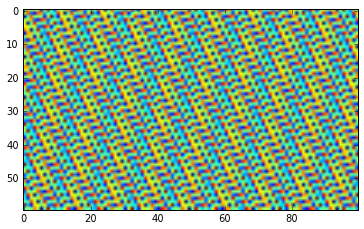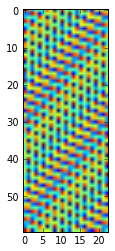The second image does not immediately appear to be a subsample of the first.

A simple image template matching algorithm slides the template over the image comparing each overlap. (Here I will be using the `match_template` function in `skimage.feature` which does essentially this.) Image template matching algorithms that work in this manner would not recognize the above smaller image inside the larger one.

In :
```from skimage.feature import match_template
print match_template.__doc__[:340]
```
```Match a template to an image using normalized correlation.

The output is an array with values between -1.0 and 1.0, which correspond
to the probability that the template is found at that position.

Parameters
----------
image : array_like
Image to process.
template : array_like
Template to locate.

```
In :
```result = match_template(full_image, sample_image)
result.max()
```
Out:
```0.019117253
```

As you can see `match_template` gives a very low probability of the template being anywhere in the image.

## Enter the Fourier Transform

This large sensitivity to time shifts can be mitigated by moving out of the time domain and into the frequency domain. This can be accomplished by using the Discrete Fourier Transform, and it can be accomplished quickly by using the Fast Fourier Transform. (For a good discussion of the DFT and FFT see this blog post by Jake VanderPlas)

If we take the fourier transform of each column in our reshaped signal, we can get a feel for how the frequency components change over time. It turns out this is a common technique and is known as a spectrogram.

In a nutshell the fourier transform tells us which frequencies have the highest energies in a time domain signal. Since the frequency components in an audio signal usually change over time, the spectrogram combines the nice properties of both the time and frequency domains.

Lets see a spectrogram of the dummy audio data:

In :
```def spectrogram(data, segment_size=60):
end = len(data) - len(data) % segment_size
stacked = data[:end].reshape((-1, segment_size))
freq_space = np.fft.fft(stacked)
real = np.abs(freq_space)
# fft results are mirrored, this trims the excess
trimmed = real.T[:segment_size/2, :]
return trimmed

spec = spectrogram(audio_data)
imshow(spec)
```
Out:
```<matplotlib.image.AxesImage at 0x11174bdd0>
```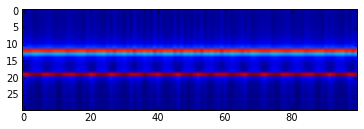As you can see there are two prominent horizontal bands. This indicates the audio signal consists of the combination of two frequencies that do not change over time. This makes sense of course because we generated the dummy audio data simply by summing two sine waves of difference frequencies.

Now we have something that looks like an image and in theory corresponds well with time shifted audio sub samples. Let's test that theory:

In :
```sample_spec = spectrogram(audio_sample)
result = match_template(spec, sample_spec)
result.max()
```
Out:
```1.0000006
```

Success! `match_template` is telling us that these two "images" are highly correlated. However, does it work with audio samples that are more than just the sum of two sine waves?

## Real Data

I ultimately want to use my computer to detect which episode of a given TV series is currently playing. Here I will be using Adventure Time Season 1. I have 11 WAV files (one for each episode) in a subdirectory `adv_time`.

In :
```from scipy.io import wavfile
audio = np.sum(audio, 1)  #sum the channels
sample = audio[10000000:10200000]  # ~4.5 second subsample
spec = spectrogram(audio, segment_size=512)
sample_spec = spectrogram(sample, segment_size=512)
imshow(sample_spec)
```
Out:
```<matplotlib.image.AxesImage at 0x114eb8090>
```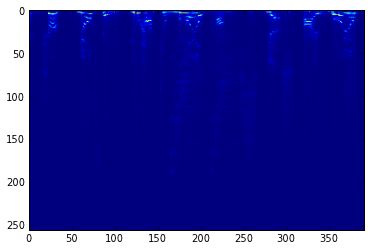In :
```%timeit result = match_template(spec, sample_spec)
```
```1 loops, best of 3: 18 s per loop

```
In :
```plot(result[0,:])  # plot 1 dim as a line
```
Out:
```[<matplotlib.lines.Line2D at 0x115184050>]
```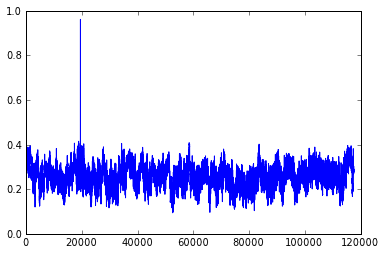A peak in the result! `match_template` is fairly confident that the subsample is part of the original audio data. However the template matching algorithm took about 20 seconds to run, which is entirely too long. We will need to lose some data to speed this up.

A man named Nyquist taught us all that the highest frequency we can reliably detect in a signal is equal to one half the sampling rate. This is known as the Nyquist Frequency. The sampling rate of our data was returned by `wavefile.read()`:

In :
```sampling_rate
```
Out:
```44100
```

A sampling rate of 44.1 kHz means we can detect audio frequencies up to 22 kHz. This is convenient since the upper limit on human hearing is around 20 kHz

However, looking at the spectrum above it seems like almost all of the interesting bits are in the top half, and a large number of them are in the top eighth. It would seem that use useful information embedded in the audio track of Adventure Time is not close to the upper limit of human perception. This implies we can resample the audio by a factor of 8 and not lose too much information.

In :
```downsampled = audio.reshape((-1, 8)).mean(1)
downsampled_sample = sample.reshape((-1, 8)).mean(1)
spec = spectrogram(downsampled, segment_size=512)
sample_spec = spectrogram(downsampled_sample, segment_size=512)
result = match_template(spec, sample_spec)
print result.max()
```
```0.714121

```
In :
```%timeit match_template(spec, sample_spec)
```
```1 loops, best of 3: 1.23 s per loop

```
In :
```plot(result[0,:])
```
Out:
```[<matplotlib.lines.Line2D at 0x126972c10>]
```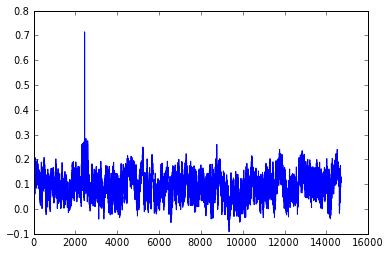Much better. The downsampling awarded us a factor of ~15 speedup while still giving plenty of signal above the noise. Even though the highest likelihood of a match is only 71%, that is still much higher than the surrounding noise floor. However I still want to drop some data to speed up the matching. If we downsample any more in the time domain we will really begin to lose important higher frequency information, but we can still try to downsample in the frequency domain.

In :
```def downsample2d(a, factor):
e0 = a.shape - a.shape % factor
e1 = a.shape - a.shape % factor
shape = a.shape / factor, a.shape / factor
sh = shape, a.shape//shape, shape, a.shape//shape
return a[:e0, :e1].reshape(sh).mean(-1).mean(1)

down_spec = downsample2d(spec, 2)
down_sample_spec = downsample2d(sample_spec, 2)

result = match_template(down_spec, down_sample_spec)
plot(result[0,:])
```
Out:
```[<matplotlib.lines.Line2D at 0x11110bf50>]
```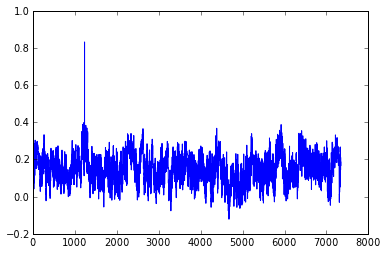In :
```%timeit match_template(down_spec, down_sample_spec)
```
```1 loops, best of 3: 278 ms per loop

```

Another factor of 4 speed up while still maintaining a good signal to noise ratio! At this point I am happy enough with the speed. With the downsampling I can compare a ~5 second sub sample to about two hours of audio in one second. But there is still one final test. So far we have only been running the tests using exact sub samples. I need to find out if this can work with samples taken using my computer's microphone.

Below is the code I used to run this experiment. First I preprocessed the audio for each episode and stored them in a dictionary called `store`. I then used `pyaudio` on my laptop to record the audio playing from an episode of Adventure Time on my TV.

In :
```import os
import pyaudio

def process_wavfile(filename, store):
""" Open the given wavfile, downsample it, compute the
spectrogram, and downsample again. Store the result in
the given `store` keyed under the filename.
"""
name = filename.split('/')[-1].split('.')
downsampled = audio.reshape((-1, 16)).mean(1)
spec = spectrogram(downsampled, segment_size=512)
down_spec = downsample2d(spec, 2)
store[name] = down_spec

def acquire_audio(seconds=5):
""" Acquire audio for the given duration.
"""
rate = 11025
chunk = 1024
p = pyaudio.PyAudio()
stream = p.open(format=pyaudio.paInt16,
channels=1,
rate=rate,
input=True,
frames_per_buffer=chunk)
frames = []
for _ in range(int(rate / chunk * seconds)):
stream.stop_stream()
stream.close()
p.terminate()

ary = np.fromstring(b''.join(frames), dtype=np.short)
ary = ary.reshape((-1, 2)).mean(1)
return ary

def process_acquired(ary):
""" Calculate the spectrogram and downsample the
given audio array.
"""
spec = spectrogram(ary, segment_size=512)
down_spec = downsample2d(spec, 2)
return down_spec

store = {}
```
In :
```acquired = acquire_audio(5)
processed = process_acquired(acquired)

results = {}
for name, signature in store.iteritems():
result = match_template(signature, processed)
results[name] = result

top = sorted(results.items(), key=lambda i: i.max(), reverse=True)
for name, result in top[:3]:  # print the top three matches
print name, result.max()
```
```ep1 0.750591
ep8 0.458766
ep10 0.440036

```
In :
```for name, result in results.iteritems():
plot(result[0, :])
```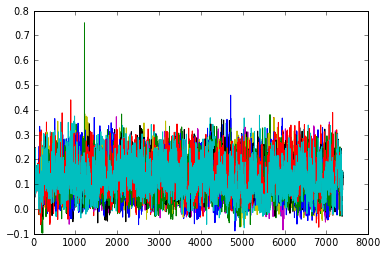It works! As you can see in the printed scores and the plot there is a strong peak for episode one, which is the episode I was playing at the time. This code was able to successfully identify which episode of Adventure Time (11 episodes total) I was watching with a high likelihood in under 3 seconds.

## Conclusion

I set out to use image processing algorithms in domains other than image processing. I ended up with a successful application in audio fingerprinting. This may not be the best way to tackle the audio fingerprinting problem (I am sure Shazaam has a much more performant algorithm) but it was fun to explore. I tried using other template matching approaches such as Harris Corner Detection and SURF (See github), but this simple approach was the most robust and easy to implement. Further work might explore those routes more.

I didn't write memory effecient numpy code, and I imagine some more performance could be eeked out there. Along with memory optimizations these algorithms have many knobs to twist, and I did not spend much time trying to optimize them. Future work might explore the sweet spot of downsampling in both the time and frequency domains. I would also like to play with the tradeoff between sample length in time and downsampling.

I would like to explore the robustness of this algorithm in the face of varying audio sources. My downsampling might not have been detrimental to the specific type of audio I was using, but may have ruined detection in another source.

If you have questions, suggestions, ideas or complaints please reach out to me on twitter: jackminardi

You can find all the code on github

Follow the discussion on Hacker News

This blog post was written entirely in an IPython Notebook. You can download the full notebook here